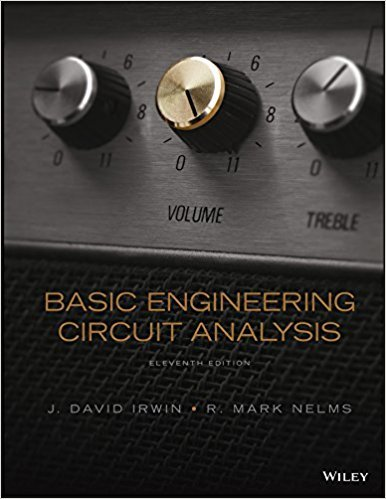×
Get Full Access to Basic Engineering Circuit Analysis - 11 Edition - Chapter 6 - Problem 6.25
Get Full Access to Basic Engineering Circuit Analysis - 11 Edition - Chapter 6 - Problem 6.25

×

# The voltage across a 10-mH inductor is shown in Fig.ISBN: 9781118539293 159

## Solution for problem 6.25 Chapter 6

Basic Engineering Circuit Analysis | 11th Edition

• Textbook Solutions
• 2901 Step-by-step solutions solved by professors and subject experts
• Get 24/7 help from StudySoup virtual teaching assistantsBasic Engineering Circuit Analysis | 11th Edition

4 5 1 413 Reviews
16
2
Problem 6.25

The voltage across a 10-mH inductor is shown in Fig. P6.25. Determine the waveform for the inductor current. 0 1 2 10 (t) (mV) t (ms) Figure P6.25

Step-by-Step Solution:
Step 1 of 3

Lab #1 The Penny Problem: Review The Scientific Method Scientists use the scientific method to answer questions and provide explanations about natural phenomena. It is a logical process based on careful observation and experimentation. 1. Observation: leads to a question and discussion about the observed phenomena. 2. Hypothesis: based on the observation scientists generate a hypothesis or tentative explanation for the observed phenomena. Hypothesis are written as two statements; one called the null hypothesis, and the other called alternate hypotheses. - The Null Hypothesis – is written in a way that shows no difference between the groups under study. Ex: There is statistically significant relationship between the color of pennies and their weight. - The Alternate Hypothesis – is written in a way that shows how a “successful” difference will be found. Ex: There is a statistically significant relationship between the color of the pennies and their weight. - A good hypothesis is testable and offers only one explanation. In addition, predictions are used for the creation of carefully designed experiments. 3. Experiments – should only test one factor (variable) at a time with all other conditions being kept constant. 4 Results – Data collected from the experiments are analyzed using statistics, are evaluated in respect to the original hypothesis statement. It is important to note that there are two types of data: Continuous: All possible and available numeric outcomes. Measurements of weights, with accurate electronic balances it is possible to measure 1.0, 1.01, and 1.001 grams. Group sample means (averages) are then determined. Scalar: “either / or”, “heads or tails”, “male or female”, etc. - Group sample medians are then determined. From the statistical analysis, conclusions are drawn. 5. Conclusion – If the experiments has been designed properly, the data should support or reject the hypotheses. Note: If you can neither support nor reject the hypothesis statement, then the experiment has been poorly designed. If the experiments analysis leads to a rejection of the alternate hypothesis (null hypothesis accepted), then a new or revised hypothesis statement is constructed and the process starts over. If the hypothesis is supported (alternate hypothesis accepted), further experimentation is still warranted because repetition will always strengthen the evidence for acceptance of the alternate hypothesis. 6. A Frequency Histogram - Plots mass in tenths (or one one­hundredth) of a gram on the “X” (horizontal) axis (this is the independent variable) - “Y” axis is used to record each individual outcome having a particular mass value (this is the dependent variable) Samples – groups taken out of a population What is the difference between a sample and a population - Sample: specific group - Population: includes all groups

Step 2 of 3

Step 3 of 3

##### ISBN: 9781118539293

Unlock Textbook Solution